• 使用LSTM进行时间序列预测 欢迎使用Markdown编辑器 你好！ 这是你第一次使用 Markdown编辑器 所展示的欢迎页。如果你想学习如何使用Markdown编辑器, 可以仔细阅读这篇文章，了解一下Markdown的基本语法知识。 新...
简单粗暴LSTM
LSTM进行时间序列预测
示例数据下载
https://download.csdn.net/download/weixin_40066612/13714885?utm_source=bbsseo
此数据是1949 到 1960 一共 12 年，每年 12 个月的航班乘客数据，一共 144 个数据，单位是 1000。我们使用它来进行LSTM时间序列预测的实验。
数据如图所示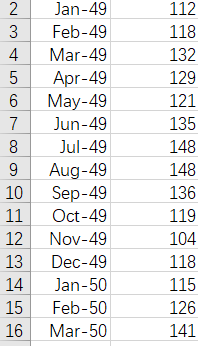第一列为时间 第二列为数据
编写代码
头文件
import numpy
import matplotlib.pyplot as plt
from keras.models import Sequential
from keras.layers import Dense
from keras.layers import LSTM
import  pandas as pd
import  os
from keras.models import Sequential, load_model

加载数据
在这里我们设置时序数据的前65%为训练数据  后35%为测试数据
dataframe = pd.read_csv('./international-airline-passengers.csv', usecols=, engine='python', skipfooter=3)
dataset = dataframe.values
# 将整型变为float
dataset = dataset.astype('float32')
#归一化 在下一步会讲解
scaler = MinMaxScaler(feature_range=(0, 1))
dataset = scaler.fit_transform(dataset)

train_size = int(len(dataset) * 0.65)
trainlist = dataset[:train_size]
testlist = dataset[train_size:]

对数据进行处理
LSTM进行预测需要的是时序数据 根据前timestep步预测后面的数据
假定给一个数据集
{
A,B,C->D
B,C,D->E
C,D,E->F
D,E,F->G
E,F,G->H
}
这时timestep为3，即根据前三个的数据预测后一个数据的值
所以我们需要对数据进行转化
举一个简单的情况  假设一个list为[1,2,3,4,5],timestep = 2
我们转化之后要达到的效果是

train_X
train_Y

[1,2]


[2,3]


[3,4]


即依据前两个值预测下一个值

对数据进行归一化
LSTM可以不进行归一化的操作，但是这样会让训练模型的loss下降很慢。本教程如果不进行归一化，100次迭代后loss还是很高
#上面代码的片段讲解
scaler = MinMaxScaler(feature_range=(0, 1))
dataset = scaler.fit_transform(dataset)

对数据进行处理
def create_dataset(dataset, look_back):
#这里的look_back与timestep相同
dataX, dataY = [], []
for i in range(len(dataset)-look_back-1):
a = dataset[i:(i+look_back)]
dataX.append(a)
dataY.append(dataset[i + look_back])
return numpy.array(dataX),numpy.array(dataY)
#训练数据太少 look_back并不能过大
look_back = 1
trainX,trainY  = create_dataset(trainlist,look_back)
testX,testY = create_dataset(testlist,look_back)

LSTM模型
LSTM的输入为 [samples, timesteps, features]
这里的timesteps为步数，features为维度 这里我们的数据是1维的
trainX = numpy.reshape(trainX, (trainX.shape, trainX.shape, 1))
testX = numpy.reshape(testX, (testX.shape, testX.shape ,1 ))

# create and fit the LSTM network
model = Sequential()
model.add(LSTM(4, input_shape=(None,1)))
model.add(Dense(1))
model.compile(loss='mean_squared_error', optimizer='adam')
model.fit(trainX, trainY, epochs=100, batch_size=1, verbose=2)
model.save(os.path.join("DATA","Test" + ".h5"))
# make predictions

进行预测
#model = load_model(os.path.join("DATA","Test" + ".h5"))
trainPredict = model.predict(trainX)
testPredict = model.predict(testX)

#反归一化
trainPredict = scaler.inverse_transform(trainPredict)
trainY = scaler.inverse_transform(trainY)
testPredict = scaler.inverse_transform(testPredict)
testY = scaler.inverse_transform(testY)

查看结果
plt.plot(trainY)
plt.plot(trainPredict[1:])
plt.show()
plt.plot(testY)
plt.plot(testPredict[1:])
plt.show()

这个时候我们的结果为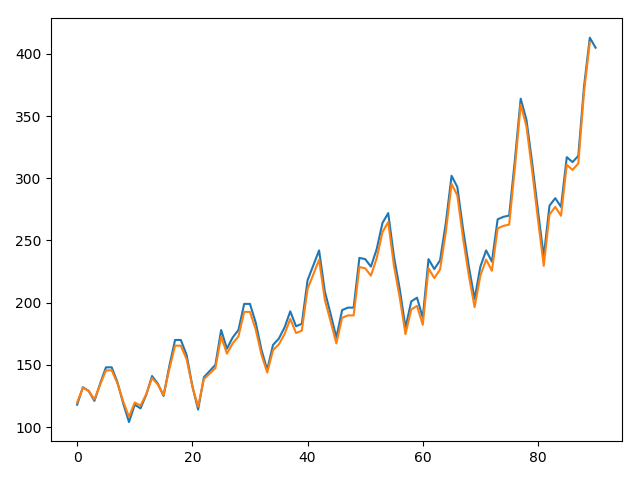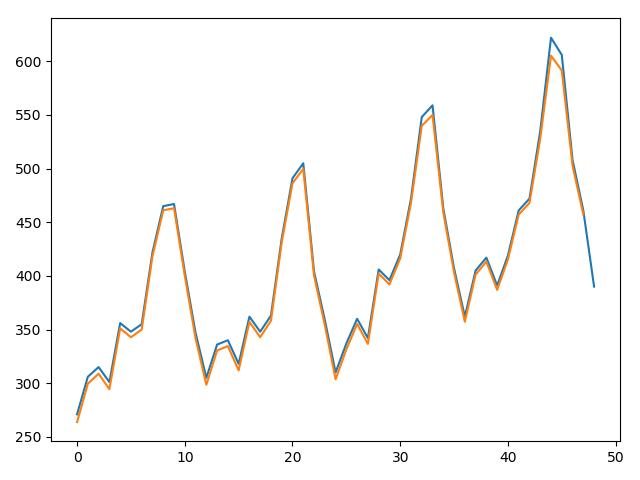参考
用 LSTM 做时间序列预测的一个小例子
Keras中文文档-Sequential model
注：代码已上传


展开全文LSTM 神经网络
• 时间序列预测的需求不仅存在于各类业务场景当中，而且通常需要对未来几年甚至几分钟之后的时间序列进行预测。如果你正要着手进行时间序列预测，那么本文将带你快速掌握一些必不可少的概念。 目录 什么是时间序列...
本文介绍了用Python进行时间序列分解的不同方法，以及如何在Python中进行时间序列预测的一些基本方法和示例。预测是一件复杂的事情，在这方面做得好的企业会在同行业中出类拔萃。时间序列预测的需求不仅存在于各类业务场景当中，而且通常需要对未来几年甚至几分钟之后的时间序列进行预测。如果你正要着手进行时间序列预测，那么本文将带你快速掌握一些必不可少的概念。

目录

什么是时间序列？

如何在Python中绘制时间序列数据？

时间序列的要素是什么？

如何分解时间序列？

经典分解法

如何获得季节性调整值？

STL分解法

时间序列预测的基本方法：

Python中的简单移动平均（SMA）

为什么使用简单移动平均？

Python中的加权移动平均（WMA）

Python中的指数移动平均（EMA）

什么是时间序列？

顾名思义，时间序列是按照固定时间间隔记录的数据集。换句话说，以时间为索引的一组数据是一个时间序列。请注意，此处的固定时间间隔（例如每小时，每天，每周，每月，每季度）是至关重要的，意味着时间单位不应改变。别把它与序列中的缺失值混为一谈。我们有相应的方法来填充时间序列中的缺失值。

在开始使用时间序列数据预测未来值之前，思考一下我们需要提前多久给出预测是尤其重要的。你是否应该提前一天，一周，六个月或十年来预测（我们用“界限”来表述这个技术术语）？需要进行预测的频率是什么？在开始预测未来值的详细工作之前，与将要使用你的预测结果的人谈一谈也不失为一个好主意。

如何在PYTHON中绘制时间序列数据？

可视化时间序列数据是数据科学家了解数据模式，时变性，异常值，离群值以及查看不同变量之间的关系所要做的第一件事。从绘图查看中获得的分析和见解不仅将有助于建立更好的预测，而且还将引导我们找到最合适的建模方法。这里我们将首先绘制折线图。折线图也许是时间序列数据可视化最通用的工具。

这里我们用到的是AirPassengers数据集。该数据集是从1949年到1960年之间的每月航空旅客人数的集合。下面是一个示例数据，以便你对数据信息有个大概了解。

#Reading Time Series DataAirpassenger = pd.read_csv("AirPassengers.csv")Airpassenger.head(3)

现在，我们使用折线图绘制数据。在下面的示例中，我们使用set_index（）将date列转换为索引。这样就会自动在x轴上显示时间。接下来，我们使用rcParams设置图形大小，最后使用plot（）函数绘制图表。

Airpassenger = Airpassenger.set_index('date')pyplot.rcParams["figure.figsize"] = (12,6)Airpassenger.plot()pyplot.show()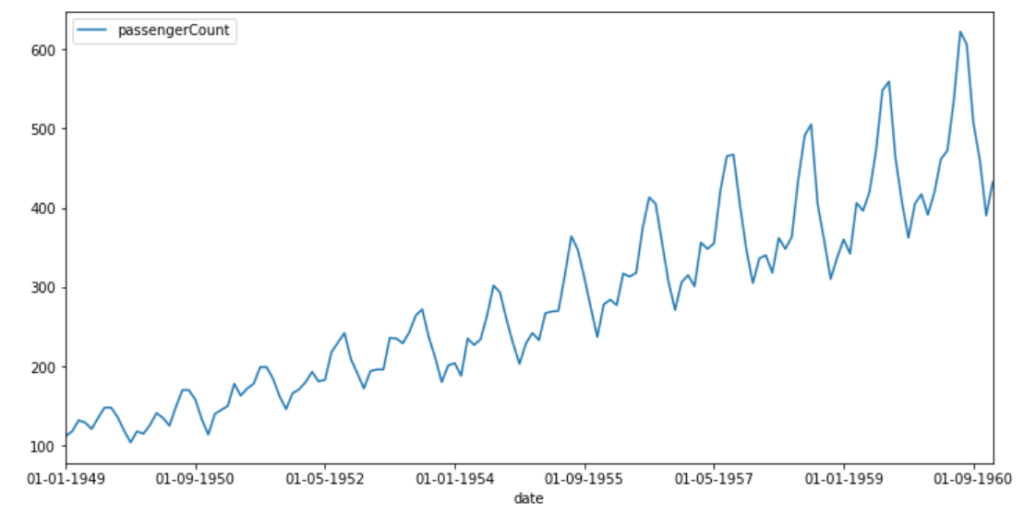航空旅客人数

1949-1960年间，乘飞机旅行的乘客人数稳定增长。规律性间隔的峰值表明增长似乎在有规律的时间间隔内重复。

让我们看看每个季度的趋势是怎样的。为了便于理解，从不同的维度观察信息是个好主意。为此，我们需要使用Python中的datetime包从date变量中得出季度和年份。在进行绘图之前，我们将连接年份和季度信息，以了解旅客数量在季节维度上如何变化。

from datetime import datetime# Airpassenger["date"] = Airpassenger["date"].apply(lambda x: datetime.strptime(x, "%d-%m-%Y"))Airpassenger["year"] = Airpassenger["date"].apply(lambda x: x.year)Airpassenger["qtr"] = Airpassenger["date"].apply(lambda x: x.quarter)Airpassenger["yearQtr"]=Airpassenger['year'].astype(str)+'_'+Airpassenger['qtr'].astype(str)airPassengerByQtr=Airpassenger[["passengerCount", "yearQtr"]].groupby(["yearQtr"]).sum()

准备好绘制数据后，我们绘制折线图，并确保将所有时间标签都放到x轴。x轴的标签数量非常多，因此我们决定将标签旋转呈现。

pyplot.rcParams["figure.figsize"] = (14,6)pyplot.plot(airPassengerByQtr)pyplot.xticks(airPassengerByQtr.index, rotation='vertical')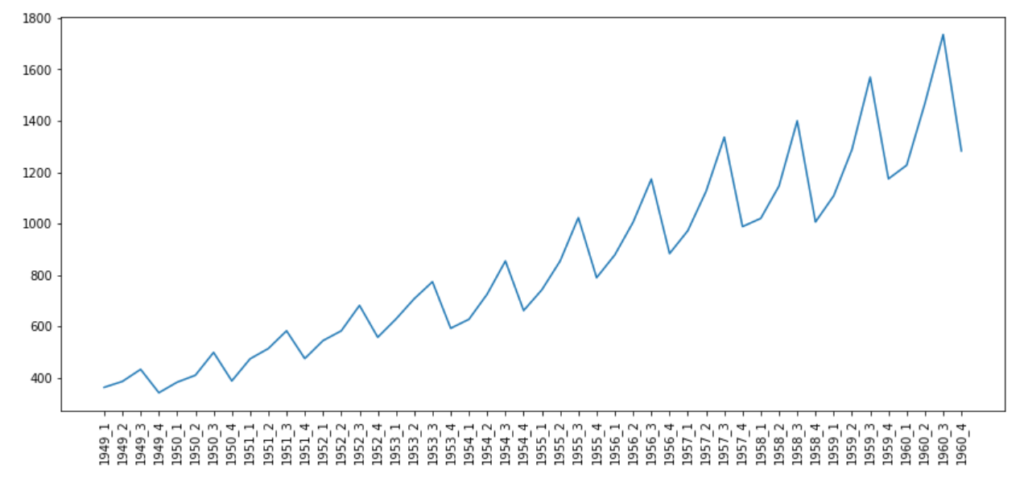每季度的旅客总数

这幅图非常有趣，它清晰地表明，在1949-1960年之间的所有年份中，航空旅客人数每季度都在显著增加。

时间序列的要素是什么？

时间序列数据包含4个主要元素：

1. 趋势性–趋势性表示数据随时间增加或减少的一般趋势。这很容易理解。例如，1949年至1960年之间航空旅客数量呈增加趋势，或者可以说呈上升趋势。

2. 季节性–如同一年四季，数据模式出现在有规律的间隔之后，代表了时间序列的季节性组成部分。它们在特定的时间间隔（例如日，周，月，年等）之后重复。有时我们很容易弄清楚季节性，有时则未必。通常，我们可以绘制图表并直观检验季节性元素的存在。但是有时，我们可能不得不依靠统计方法来检验季节性。

3. 周期性–可被视为类似季节性，但唯一的区别是周期性不会定期出现。这个属性使得它很难被辨识。例如，地震可以在我们知道将要发生的任何时间发生，但是我们其实不知道何时何地发生。

4. 随机噪声–不属于上述三类情况的时间序列数据中的突然变化，而且也很难被解释，因此被称为随机波动或随机噪声。

如何分解时间序列？

有两种技术可以获取时间序列要素。在进行深入研究和查看相关Python抽取函数之前，必须了解以下两点：

时间序列不必具有所有要素。

弄清该时间序列是可加的还是可乘的。

那么什么是可加和可乘时间序列模型呢？

可加性模型–在可加性模型中，要素之间是累加的关系。

y（t）=季节+趋势+周期+噪音

可乘性模型–在可乘性模型中，要素之间是相乘的关系。

y（t）=季节*趋势*周期*噪音

你想知道为什么我们还要分解时间序列吗？你看，分解背后的目的之一是估计季节性影响并提供经过季节性调整的值。去除季节性的值就可以轻松查看趋势。例如，在美国，由于农业领域需求的增加，夏季的失业率有所下降。从经济学角度来讲，这也意味着6月份的失业率与5月份相比有所下降。现在，如果你已经知道了逻辑，这并不代表真实的情况，我们必须调整这一事实，即6月份的失业率始终低于5月份。

这里的挑战在于，在现实世界中，时间序列可能是可加性和可乘性的组合。这意味着我们可能并不总是能够将时间序列完全分解为可加的或可乘的。

现在你已经了解了不同的模型，下面让我们研究一些提取时间序列要素的常用方法。

经典分解法

该方法起源于1920年，是诸多方法的鼻祖。经典分解法有两种形式：加法和乘法。Python中的statsmodels库中的函数season_decompose（）提供了经典分解法的实现。在经典分解法中，需要你指出时间序列是可加的还是可乘的。你可以在此处（https://otexts.com/fpp2/classical-decomposition.html）了解有关加法和乘法分解的更多信息。

在下面的代码中，要获得时间序列的分解，只需赋值model=additive。

import numpy as npfrom pandas import read_csvimport matplotlib.pyplot as pltfrom statsmodels.tsa.seasonal import seasonal_decomposefrom pylab import rcParams elecequip = read_csv(r"C:/Users/datas/python/data/elecequip.csv")result = seasonal_decompose(np.array(elecequip), model='multiplicative', freq=4) rcParams['figure.figsize'] = 10, 5result.plot()pyplot.figure(figsize=(40,10))pyplot.show()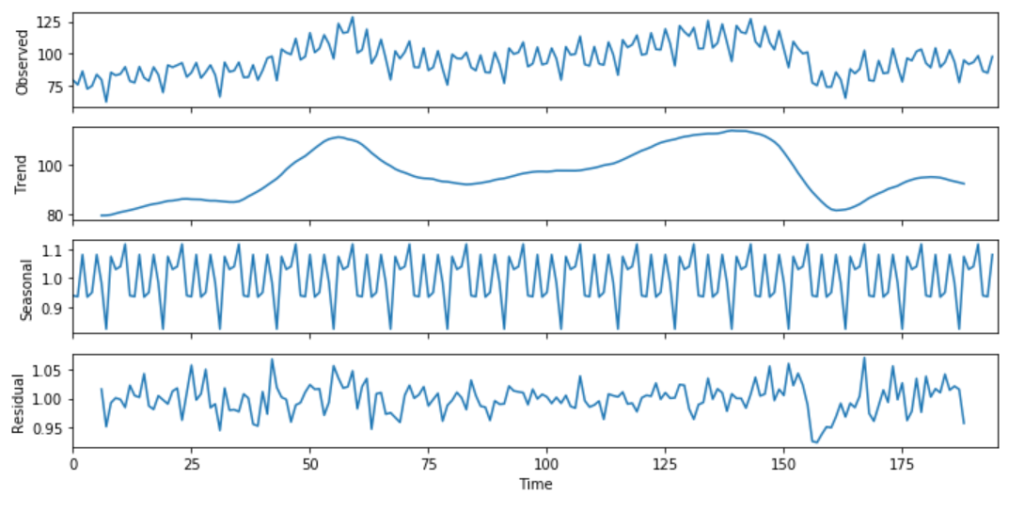上图的第一行代表实际数据，底部的三行显示了三个要素。这三个要素累加之后即可以获得原始数据。第二个样本集代表趋势性，第三个样本集代表季节性。如果我们考虑完整的时间范围，你会看到趋势一直在变化，并且在波动。对于季节性，很明显，在规律的时间间隔之后可以看到峰值。

如何获得季节性调整值？

对于可加性模型，可以通过y（t）– s（t）获得季节性调整后的值，对于乘法数据，可以使用y（t）/ s（t）来调整值。

如果你正想问为什么我们需要季节性调整后的数据，让我们回顾一下刚才讨论过的有关美国失业率的示例。因此，如果季节性本身不是我们的主要关注点，那么季节性调整后的数据将更有用。尽管经典方法很常见，但由于以下原因，不太建议使用它们：

该技术对异常值不可靠。

它倾向于使时间序列数据中的突然上升和下降过度平滑。

假设季节性因素每年只重复一次。

对于前几次和最后几次观察，该方法都不会产生趋势周期估计。

其他可用于分解的更好方法是X11分解，SEAT分解或STL分解。现在，我们将看到如何在Python中生成它们。

与经典法，X11和SEAT分解法相比，STL具有许多优点。接下来，让我们探讨STL分解法。

STL分解法

STL代表使用局部加权回归（Loess）进行季节性和趋势性分解。该方法对异常值具有鲁棒性，可以处理任何类型的季节性。这个特性还使其成为一种通用的分解方法。使用STL时，你控制的几件事是：

趋势周期平滑度

季节性变化率

可以控制对用户异常值或异常值的鲁棒性。这样你就可以控制离群值对季节性和趋势性的影响。

同任何其他方法一样，STL也有其缺点。例如，它不能自动处理日历的变动。而且，它仅提供对可加性模型的分解。但是你可以得到乘法分解。你可以首先获取数据日志，然后通过反向传播要素来获取结果。但是，这超出了本文讨论的范围。

Import pandas as pdImport seaborn as snsImport matplotlib.pyplot as pltFrom statsmodels.tsa.seasonal import STL
elecequip =read_csv(r"C:/Users/datas/python/data/elecequip.csv")stl = STL(elecequip, period=12, robust=True)res_robust = stl.fit()fig = res_robust.plot()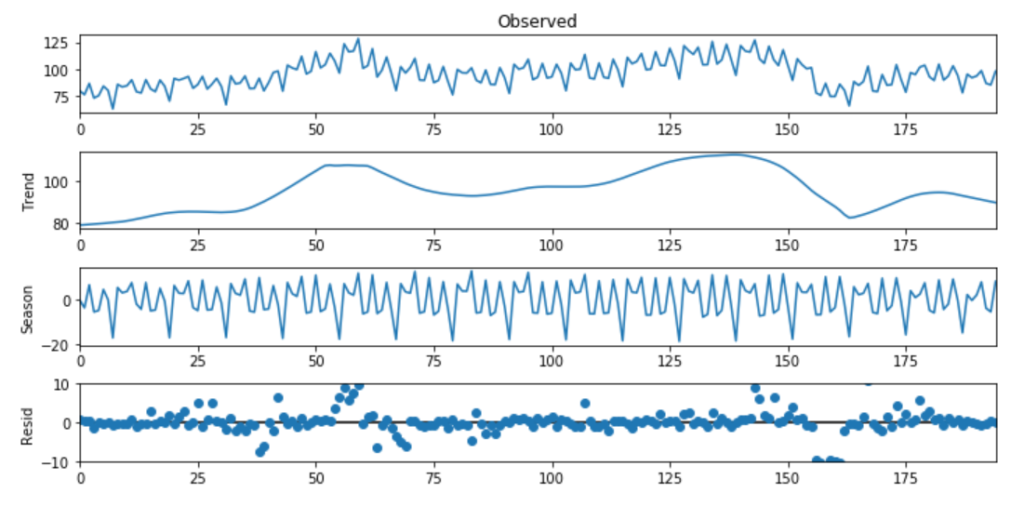时间序列预测的基本方法

尽管有许多统计技术可用于预测时间序列数据，我们这里仅介绍可用于有效的时间序列预测的最直接、最简单的方法。这些方法还将用作其他方法的基础。

PYTHON中的简单移动平均（SMA）

简单移动平均是可以用来预测的所有技术中最简单的一种。通过取最后N个值的平均值来计算移动平均值。我们获得的平均值被视为下一个时期的预测。

为什么使用简单移动平均？

移动平均有助于我们快速识别数据趋势。你可以使用移动平均值确定数据是遵循上升趋势还是下降趋势。它可以消除波峰波谷等不规则现象。这种计算移动平均值的方法称为尾随移动平均值。在下面的示例中，我们使用rolling（）函数来获取电气设备销售数据的移动平均线。

Import pandas as pdfrom matplotlib import pyplot elecequip = pd.read_csv(r"C:/Users/datas/python/data/elecequip.csv") # Taking moving average of last 6 obsrolling = elecequip.rolling(window=6)rolling_mean = rolling.mean() # plot the two seriespyplot.plot(elecequip)pyplot.plot(rolling_mean, color='red')pyplot.show()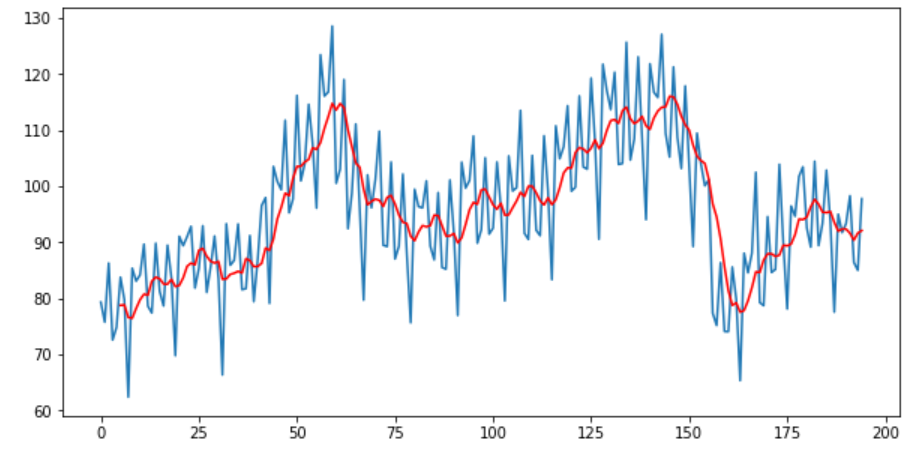另一种方法是“中心移动平均”。在这里将任意给定时间（t）的值计算为当前，之前和之后的平均值。启用center = True将提供中心移动平均值。

elecequip["x"].rolling(window=3, center=True).mean()

PYTHON中的加权移动平均（WMA）

简单移动平均非常朴素，因为它对过去的所有值给予同等的权重。但是当假设最新数据与实际值密切相关，则对最新值赋予更多权重可能更有意义。

要计算WMA，我们要做的就是将过去的每个观察值乘以一定的权重。例如，在6周的滚动窗口中，我们可以将6个权重赋给最近值，将1个权重赋给最后一个值。

import randomrand = [random.randint(1, i) for i in range(100,110)]data = {}data["Sales"] = rand
df = pd.DataFrame(data)weights = np.array([0.5, 0.25, 0.10])
sum_weights = np.sum(weights)df['WMA']=(df['Sales'].rolling(window=3, center=True).apply(lambda x: np.sum(weights*x)/sum_weights, raw=False)
)print(df['WMA'])

PYTHON中的指数移动平均（EMA）

在“指数移动平均”中，随着观察值的增加，权重将按指数递减。该方法通常是一种出色的平滑技术，可以从数据中消除很多噪声，从而获得更好的预测。

import numpy as npimport pandas as pdimport matplotlib.pyplot as pltfrom statsmodels.tsa.api import ExponentialSmoothing EMA_fit = ExponentialSmoothing(elecequip, seasonal_periods=12, trend='add', seasonal='add').fit(use_boxcox=True)fcast3 = EMA_fit.forecast(12)  ax = elecequip.plot(figsize=(10,6), marker='o', color='black', title="Forecasts from Exponential Smoothing" )ax.set_ylabel("Electrical Equipment")ax.set_xlabel("Index") # For plotting fitted values# EMA_fit.fittedvalues.plot(ax=ax, style='--', color='red')
EMA_fit.forecast(12).rename('EMS Forecast').plot(ax=ax, style='--', marker='o', color='blue', legend=True)

该方法具有以下两种变体：

1. 简单指数平滑–如果时间序列数据是具有恒定方差且没有季节性的可加性模型，则可以使用简单指数平滑来进行短期预测。

2. Holt指数平滑法–如果时间序列是趋势增加或减少且没有季节性的可加性模型，则可以使用Holt指数平滑法进行短期预测。

以下是从python中的statsmodels包导入两个模型的代码。现在，你可以在练习中运行上述模型。

1

2

3

4

import numpy as np

import pandas as pd

import matplotlib.pyplot as plt

from statsmodels.tsa.api import SimpleExpSmoothing, Holt

在本长篇教程中，我们讲解了：

什么是时间序列数据？

如何可视化和更深入地识别数据模式（如果有）？

介绍了可加性和可乘性时间序列模型。

研究了Python中分解时间序列的不同方法。

最后，我们学习了如何在Python中运行一些非常基本的方法，例如移动平均（MA），加权移动平均（WMA），指数平滑模型（ESM）及其变体，例如SESM和Hotl。


展开全文• 导读: 什么是基线预测，基线预测有什么呢？ 1、首先将数据按照一定的方法转换...再这个模型对未来数据进行预测。 4、最后将预测值和真实值进行残差比较，得出预测值和真实值之间的差异，或者损失，这就是一个...
导读:

什么是基线预测，基线预测有什么用呢？

1、首先将数据按照一定的方法转换为监督学习数据。

2、其次构建一个数据间的对应函数关系，也叫做数据的持久化。这种映射关系的构建往往是基于我们的经验或者对数据的预处理。

3、然后使用训练数据对模型进行训练，得到一个预测模型。再用这个模型对未来数据进行预测。

4、最后将预测值和真实值进行残差比较，得出预测值和真实值之间的差异，或者损失，这就是一个最基本的基线预测。

5、后续我们在此基础之上开发出来的预测模型，得到的残差值都不能比当前这个基线预测差，否则说明我们的预测方法是不靠谱的或者无意义的。

正文：

建立基线对任何时间序列预测问题都至关重要。

性能基线可让您了解所有其他模型对您的问题的实际执行情况。

在本教程中，您将了解如何开发持久性预测，您可以使用该预测来计算使用Python的时间序列数据集的基准性能级别。

完成本教程后，您将了解：

计算时间序列预测问题的绩效基线的重要性。
如何在Python中从头开发持久性模型。
如何从持久性模型评估预测并使用它来建立性能基线。
预测绩效基准

预测性能的基线提供了一个比较点。

它是您问题的所有其他建模技术的参考点。如果模型达到或低于基线的性能，则应该修复或放弃该技术。

用于生成预测以计算基准性能的技术必须易于实现，并且不需要特定于问题的细节。

在为预测问题建立性能基线之前，必须开发测试工具。这包括：

您打算用于训练和评估模型的数据集。
该重采样你打算技术用来估计技术（如训练/测试拆分）的性能。
您打算用于评估预测的绩效指标（例如均方误差）。
准备好之后，您需要选择一种简单的技术，您可以使用该技术进行预测并计算基准性能。

目标是尽快获得时间序列预测问题的基线性能，以便您可以更好地理解数据集并开发更高级的模型。

用于进行基线预测的良好技术的三个属性是：

简单：一种几乎不需要培训或智力的方法。
快速：一种快速实现且计算微不足道的方法来进行预测。
可重复：一种确定性的方法，意味着它在给定相同输入的情况下产生预期输出。
持久性算法（“简单”预测）

监督机器学习的最常见基线方法是零规则算法。

该算法在分类的情况下预测多数类，或在回归的情况下预测平均结果。这可以用于时间序列，但不考虑时间序列数据集中的序列相关结构。

与时间序列数据集一起使用的等效技术是持久性算法。

持久性算法使用前一时间步（t-1）的值来预测下一时间步（t + 1）的预期结果。

这满足了基线预测的上述三个条件。

为了具体化，我们将研究如何开发持久性模型并使用它来为简单的单变量时间序列问题建立基线性能。首先，我们来看看Shampoo Sales数据集。

洗发水销售数据集

该数据集描述了3年期间每月的洗发水销售数量。

单位是销售计数，有36个观察。原始数据集归功于Makridakis，Wheelwright和Hyndman（1998）。

下面是前5行数据的示例，包括标题行。

"Month","Sales"
"1-01",266.0
"1-02",145.9
"1-03",183.1
"1-04",119.3
"1-05",180.3

下面是从数据市场获取的整个数据集的图表，您可以在其中下载数据集并了解有关它的更多信息。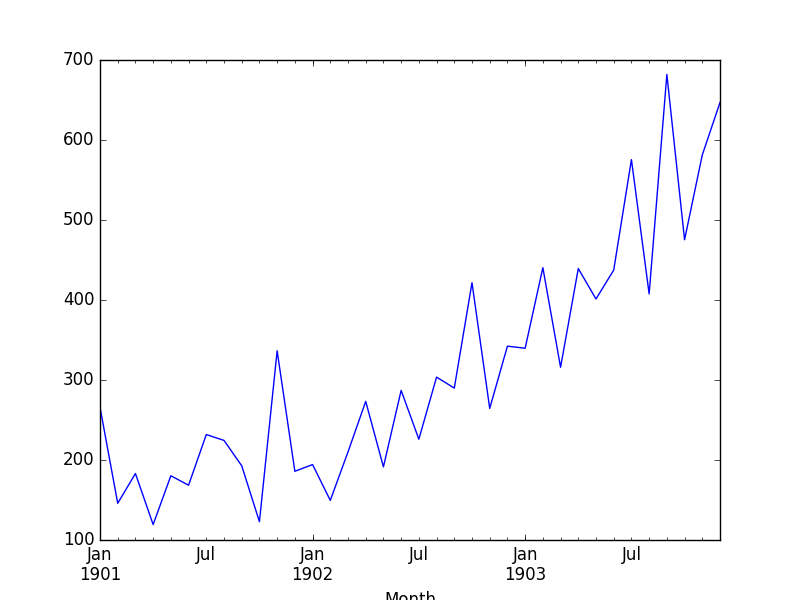洗发水销售数据集

数据集显示出增长趋势，可能还有一些季节性因素。

下载数据集并将其放在当前工作目录中，文件名为 “ shampoo-sales.csv ”。

以下代码片段将加载Shampoo Sales数据集并绘制时间序列。

from pandas import read_csv
from pandas import datetime
from matplotlib import pyplot

def parser(x):
return datetime.strptime('190'+x, '%Y-%m')

series = read_csv('shampoo-sales.csv', header=0, parse_dates=, index_col=0, squeeze=True, date_parser=parser)
series.plot()
pyplot.show()

运行该示例绘制时间序列，如下所示：洗发水销售数据集图

持久性算法

可以在Python中轻松实现持久性模型。

我们将这一部分分为4个步骤：

将单变量数据集转换为监督学习问题。
为测试工具建立训练和测试数据集。
定义持久性模型。
进行预测并建立基准性能。
查看完整示例并绘制输出。
我们潜入吧。

第1步：定义监督学习问题

第一步是加载数据集并创建滞后表示。也就是说，鉴于在t-1处的观察，预测在t + 1处的观察。

# Create lagged dataset
values = DataFrame(series.values)
dataframe = concat([values.shift(1), values], axis=1)
dataframe.columns = ['t-1', 't+1']
print(dataframe.head(5))

此代码段创建数据集并打印新数据集的前5行。

我们可以看到第一行（索引0）将不得不被丢弃，因为在第一次观察之前没有用于进行预测的观察。

从监督学习的角度来看，t-1列是输入变量，或X，而t + 1列是输出变量，或y。

t-1    t+1
0    NaN  266.0
1  266.0  145.9
2  145.9  183.1
3  183.1  119.3
4  119.3  180.3

第2步：训练和测试集

下一步是将数据集分成训练集和测试集。

我们将保留前66％的“培训”观察结果，其余34％用于评估。在拆分期间，我们小心地排除具有NaN值的第一行数据。

在这种情况下不需要培训; 这只是习惯。然后将每个列车和测试装置分成输入和输出变量。

# split into train and test sets
X = dataframe.values
train_size = int(len(X) * 0.66)
train, test = X[1:train_size], X[train_size:]
train_X, train_y = train[:,0], train[:,1]
test_X, test_y = test[:,0], test[:,1]

第3步：持久性算法

我们可以将持久性模型定义为一个返回作为输入提供的值的函数。

例如，如果提供了t6值为266.0，那么这将作为预测返回，而实际的实际值或期望值恰好是145.9（取自滞后数据集中的第一个可用行）。

# persistence model

def model_persistence(x):

return x

第4步：制作并评估预测

现在我们可以在测试数据集上评估此模型。

我们使用前向验证方法执行此操作。

不需要模型训练或再训练，因此实质上，我们逐步逐步测试数据集时间并获得预测。

一旦对训练数据集中的每个时间步进行预测，就将它们与预期值进行比较，并计算均方误差（MSE）分数。

# walk-forward validation
predictions = list()
for x in test_X:
yhat = model_persistence(x)
predictions.append(yhat)
test_score = mean_squared_error(test_y, predictions)
print('Test MSE: %.3f' % test_score)

在这种情况下，测试数据集的误差超过17,730。

Test MSE: 17730.518

第5步：完成示例

最后，绘制一个图来显示训练数据集和来自测试数据集的预期值的偏差预测。

从持久性模型预测的情节来看，很明显该模型落后于现实一步。销售数据中出现了上升趋势和月度噪音，突显了持久性技术的局限性。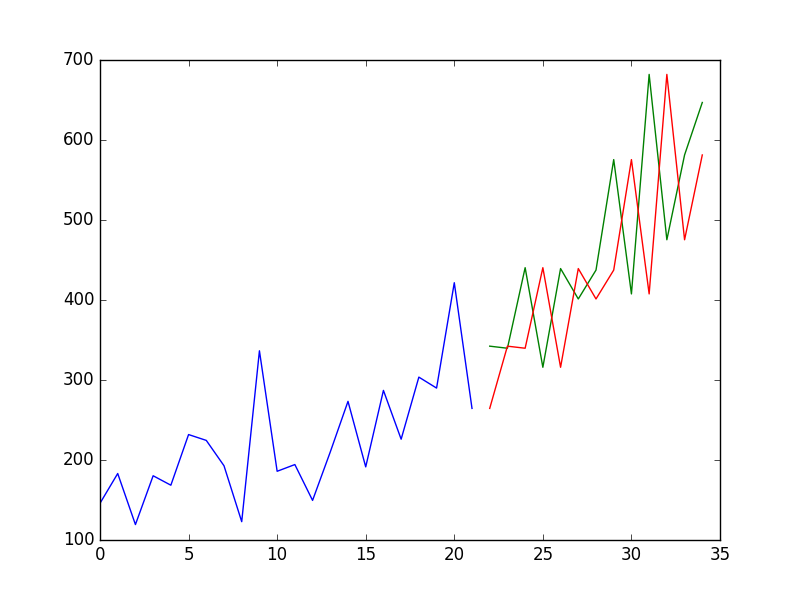洗发水销售持久性模型

下面列出了完整的示例。

from pandas import read_csv
from pandas import datetime
from pandas import DataFrame
from pandas import concat
from matplotlib import pyplot
from sklearn.metrics import mean_squared_error

def parser(x):
return datetime.strptime('190'+x, '%Y-%m')

series = read_csv('shampoo-sales.csv', header=0, parse_dates=, index_col=0, squeeze=True, date_parser=parser)
# 构建以个监督学习结构，滞后数据表
values = DataFrame(series.values)
dataframe = concat([values.shift(1), values], axis=1)
dataframe.columns = ['t-1', 't+1']
print(dataframe.head(5))

# 将数据分割为训练集和测试集
X = dataframe.values
train_size = int(len(X) * 0.66)
train, test = X[1:train_size], X[train_size:]
train_X, train_y = train[:,0], train[:,1]
test_X, test_y = test[:,0], test[:,1]

# 持久性模型，这里的映射模型是简单的对应关系
def model_persistence(x):
return x

# 用model_persistence函数模拟预测模型，对预测结果和真实值做残差比较，检验结果
predictions = list()
for x in test_X:
yhat = model_persistence(x)
predictions.append(yhat)
test_score = mean_squared_error(test_y, predictions)
print('Test MSE: %.3f' % test_score)

# 画出结果
pyplot.plot(train_y)
pyplot.plot([None for i in train_y] + [x for x in test_y])
pyplot.plot([None for i in train_y] + [x for x in predictions])
pyplot.show()

我们已经看到了从头开始为Shampoo Sales问题开发的持久性模型的示例。

持久性算法很幼稚。它通常被称为简单的预测。

它没有假设应用它的时间序列问题的具体细节。这使得它易于理解，如此快速地实施和评估。

作为机器学习从业者，它也可以激发大量的改进。

记下来。这很有用，因为这些想法可以成为特征工程工作中的输入特征，或者可以在以后的集合工作中组合的简单模型。

展开全文• Python进行时间序列预测的自回归模型 自回归是一个时间序列模型，它使用以前时间步的观察值作为回归方程的输入，以预测下一个时间步的值。... 如何开发自相关模型并用它来进行预测如何使用开发...
用Python进行时间序列预测的自回归模型

自回归是一个时间序列模型，它使用以前时间步的观察值作为回归方程的输入，以预测下一个时间步的值。

这是一个非常简单的想法，可以对一系列时间序列问题进行准确的预测。

在本教程中，您将了解如何使用Python实现时间序列预测的自回归模型。

完成本教程后，您将了解：

如何探索自相关的时间序列数据。
如何开发自相关模型并用它来进行预测。
如何使用开发的自相关模型进行滚动预测。
让我们开始吧。

自回归

回归模型（例如线性回归）基于输入值的线性组合对输出值进行建模。

例如：

yhat = b0 + b1*X1

在哪里是预测，b0和b1是通过在训练数据上优化模型而找到的系数，并且X是输入值。

该技术可用于时间序列，其中输入变量被视为前一时间步的观察值，称为滞后变量。

例如，我们可以根据最后两个时间步（t-1和t-2）的观察结果预测下一个时间步（t + 1）的值。作为回归模型，这看起来如下：

X(t+1) = b0 + b1*X(t-1) + b2*X(t-2)

因为回归模型在之前的时间步骤使用来自相同输入变量的数据，所以它被称为自回归（self of regression）。

自相关

自回归模型假设先前时间步骤的观察对于预测下一时间步的值是有用的。

变量之间的这种关系称为相关性。

如果两个变量在同一方向上变化（例如，一起向上或向下），则称为正相关。如果变量随着值的变化而向相反的方向移动（例如，一个上升，一个下降），则称为负相关。

我们可以使用统计测量来计算输出变量与先前时间步长处的各种不同滞后值之间的相关性。输出变量与特定滞后变量之间的相关性越强，自动回归模型在建模时可以对该变量赋予的权重越大。

同样，因为在先前时间步骤中在变量和它自身之间计算相关性，所以它被称为自相关。由于时间序列数据的顺序结构，它也被称为串行相关。

相关统计还可以帮助选择哪些滞后变量在模型中有用，哪些不可用。

有趣的是，如果所有滞后变量与输出变量显示低或无相关性，则表明时间序列问题可能无法预测。在开始使用新数据集时，这非常有用。

在本教程中，我们将研究单变量时间序列的自相关，然后开发自回归模型并使用它来进行预测。

在我们这样做之前，让我们首先回顾一下将在示例中使用的每日最低温度数据。

最低每日温度数据集

该数据集描述了澳大利亚墨尔本市10年（1981-1990）的最低日常温度。

单位为摄氏度，有3,650个观测值。数据来源被称为澳大利亚气象局。

在此处了解有关数据集的更多信息。

下载数据集与文件名“当前的工作目录日用最低temperatures.csv “。

注意：下载的文件包含一些问号（“？”）字符，在使用数据集之前必须删除这些字符。在文本编辑器中打开文件并删除“？”字符。同时删除文件中的任何页脚信息。

下面的代码将数据集作为Pandas系列加载。

from pandas import Series
from matplotlib import pyplot
series = Series.from_csv('daily-minimum-temperatures.csv', header=0)
print(series.head())
series.plot()
pyplot.show()

运行该示例将从加载的数据集中打印前5行。

Date
1981-01-01 20.7
1981-01-02 17.9
1981-01-03 18.8
1981-01-04 14.6
1981-01-05 15.8
Name: Temp, dtype: float64

然后创建数据集的线图。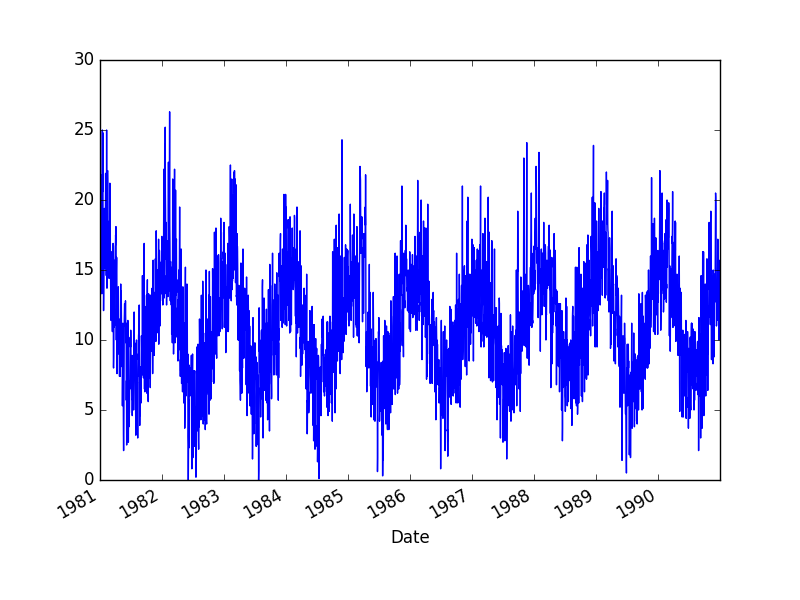最低每日温度数据集图

快速检查自相关

我们可以快速，直观地检查我们的时间序列数据集中是否存在自相关。

我们可以在前一时间步骤（t-1）绘制观察结果，并在下一时间步骤（t + 1）观察作为散点图。

这可以通过首先创建时间序列数据集的滞后版本并使用Pandas库中的内置散点图函数来手动完成。

但有一种更简单的方法。

Pandas提供了一个内置的绘图来完成这个，称为  lag_plot（）  函数。

下面是创建最低每日温度数据集的滞后图的示例。

from pandas import Series
from matplotlib import pyplot
from pandas.tools.plotting import lag_plot
series = Series.from_csv('daily-minimum-temperatures.csv', header=0)
lag_plot(series)
pyplot.show()

运行该示例将x轴上的温度数据（t）与y轴上的前一天（t-1）的温度进行对比。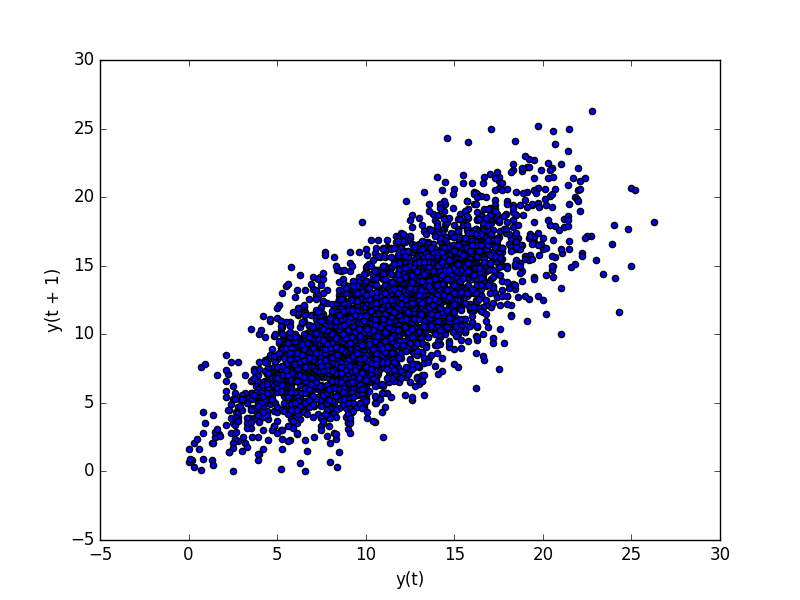最低每日温度数据集滞后图

我们可以看到沿着对角线的大型观察球。它清楚地显示了一种关系或某种相关性。

对于任何其他滞后观察，可以重复此过程，例如，如果我们想要查看过去7天或上个月或去年同一天的关系。

我们可以做的另一个快速检查是直接计算观察和滞后变量之间的相关性。

我们可以使用像Pearson相关系数这样的统计检验。这产生了一个数字，用于总结两个变量在-1（负相关）和+1（正相关）之间的相关性，其中小值接近零表示低相关性，高值高于0.5或低于-0.5表示高相关性。

可以使用滞后数据集的DataFrame上的corr（）函数轻松计算相关性。

下面的示例创建最小每日温度数据集的滞后版本，并计算每列与其他列（包括其自身）的相关矩阵。

from pandas import Series
from pandas import DataFrame
from pandas import concat
from matplotlib import pyplot
series = Series.from_csv('daily-minimum-temperatures.csv', header=0)
values = DataFrame(series.values)
dataframe = concat([values.shift(1), values], axis=1)
dataframe.columns = ['t-1', 't+1']
result = dataframe.corr()
print(result)

这是对上图的一个很好的确认。

它显示观察值与滞后= 1值之间的强正相关（0.77）。

         t-1      t+1
t-1  1.00000  0.77487
t+1  0.77487  1.00000

这对于一次性检查很有用，但如果我们想在时间序列中检查大量滞后变量，这是很乏味的。

接下来，我们将看一下此方法的扩展版本。

自相关图

我们可以绘制每个滞后变量的相关系数。

这可以非常快速地了解哪些滞后变量可能是用于预测模型的良好候选者以及观察值与其历史值之间的关系如何随时间变化。

我们可以手动计算每个滞后变量的相关值并绘制结果。值得庆幸的是，Pandas提供了一个名为autocorrelation_plot（）函数的内置图。

该图提供了沿x轴的滞后数和y轴上-1和1之间的相关系数值。该图还包括实线和虚线，表示相关值的95％和99％置信区间。这些线上方的相关值比线下方的相关值更重要，为选择更相关的滞后值提供阈值或截止值。

from pandas import Series
from matplotlib import pyplot
from pandas.tools.plotting import autocorrelation_plot
series = Series.from_csv('daily-minimum-temperatures.csv', header=0)
autocorrelation_plot(series)
pyplot.show()

运行该示例显示了正负相关的摆动，因为温度值在前一年的夏季和冬季变化。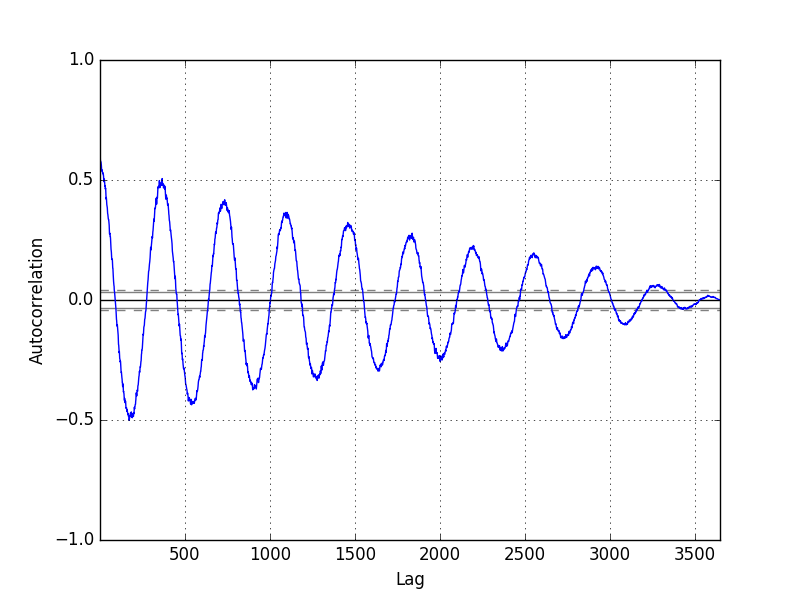熊猫自相关图

statsmodels库还在plot_acf（）函数中提供了一个版本的绘图作为线图。

from pandas import Series
from matplotlib import pyplot
from statsmodels.graphics.tsaplots import plot_acf
series = Series.from_csv('daily-minimum-temperatures.csv', header=0)
plot_acf(series, lags=31)
pyplot.show()

在此示例中，我们将评估的滞后变量限制为31以便于阅读。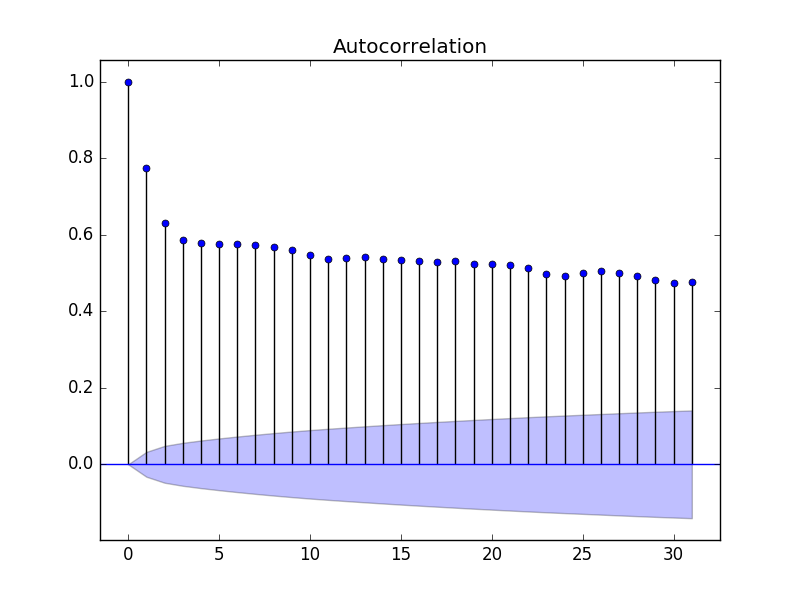Statsmodels Autocorrelation Plot

现在我们知道如何在时间序列中查看自相关，让我们看一下使用自回归建模它。

在我们这样做之前，让我们建立基准性能。

持久性模型

假设我们想要开发一个模型来预测所有先前观察结果中数据集中最近7天的最低温度。

我们可以用来做出预测的最简单模型是坚持最后一次观察。我们可以将其称为持久性模型，它为我们可用于与自回归模型进行比较的问题提供了性能基准。

我们可以通过将观察结果分成训练集和测试集来开发问题的测试工具，只将数据集中的最后7个观测值分配给测试集作为我们希望预测的“看不见的”数据。

使用前瞻性验证模型进行预测，以便我们可以保留第二天的最新观察结果。这意味着我们没有进行7天的预测，而是7天的预测。

from pandas import Series
from pandas import DataFrame
from pandas import concat
from matplotlib import pyplot
from sklearn.metrics import mean_squared_error
series = Series.from_csv('daily-minimum-temperatures.csv', header=0)
# create lagged dataset
values = DataFrame(series.values)
dataframe = concat([values.shift(1), values], axis=1)
dataframe.columns = ['t-1', 't+1']
# split into train and test sets
X = dataframe.values
train, test = X[1:len(X)-7], X[len(X)-7:]
train_X, train_y = train[:,0], train[:,1]
test_X, test_y = test[:,0], test[:,1]

# persistence model
def model_persistence(x):
return x

# walk-forward validation
predictions = list()
for x in test_X:
yhat = model_persistence(x)
predictions.append(yhat)
test_score = mean_squared_error(test_y, predictions)
print('Test MSE: %.3f' % test_score)
# plot predictions vs expected
pyplot.plot(test_y)
pyplot.plot(predictions, color='red')
pyplot.show()

运行该示例将打印均方误差（MSE）。

该值提供问题的基准性能。

Test MSE: 3.423

与模型的预测（红色）相比，绘制了接下来7天的预期值（蓝色）。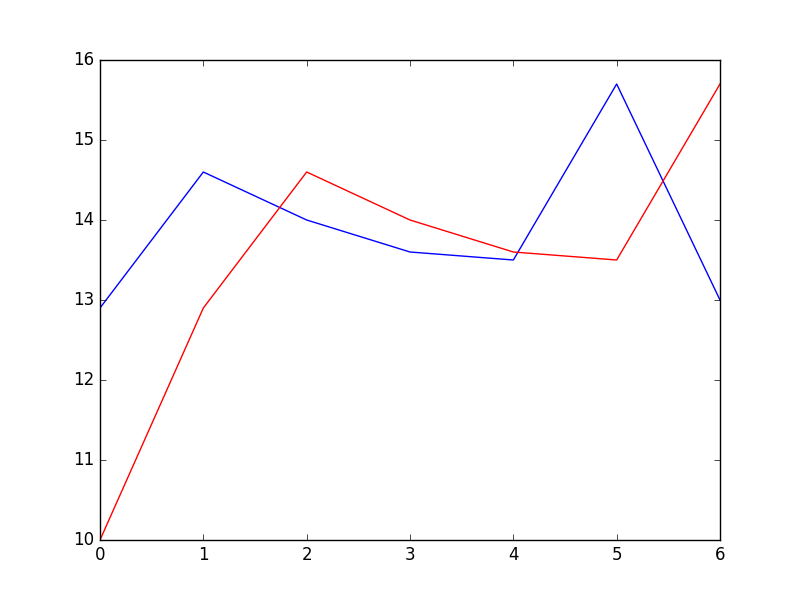持久性模型的预测

自回归模型

自回归模型是一种线性回归模型，它使用滞后变量作为输入变量。

我们可以使用scikit-learn中的LinearRegession类手动计算线性回归模型，并手动指定要使用的滞后输入变量。

或者，statsmodels库提供自动回归模型，该模型使用统计测试自动选择适当的滞后值并训练线性回归模型。它在AR类中提供。

我们可以通过首先创建模型AR（）然后调用fit（）来训练它在我们的数据集上来使用此模型。这将返回ARResult对象。

一旦适合，我们可以使用该模型通过调用future（）函数来进行预测，以用于将来的许多观察。这将创建1个7天预测，这与上面的持久性示例不同。

下面列出了完整的示例。

from pandas import Series
from matplotlib import pyplot
from statsmodels.tsa.ar_model import AR
from sklearn.metrics import mean_squared_error
series = Series.from_csv('daily-minimum-temperatures.csv', header=0)
# split dataset
X = series.values
train, test = X[1:len(X)-7], X[len(X)-7:]
# train autoregression
model = AR(train)
model_fit = model.fit()
print('Lag: %s' % model_fit.k_ar)
print('Coefficients: %s' % model_fit.params)
# make predictions
predictions = model_fit.predict(start=len(train), end=len(train)+len(test)-1, dynamic=False)
for i in range(len(predictions)):
print('predicted=%f, expected=%f' % (predictions[i], test[i]))
error = mean_squared_error(test, predictions)
print('Test MSE: %.3f' % error)
# plot results
pyplot.plot(test)
pyplot.plot(predictions, color='red')
pyplot.show()

首先运行该示例在训练的线性回归模型中打印所选择的最佳滞后和系数列表。

我们可以看到选择并训练了29个滞后模型。考虑到这个滞后与一个月的平均天数有多接近，这很有意思。

然后打印7天预测，总结预测的均方误差。

Lag: 29
Coefficients: [  5.57543506e-01   5.88595221e-01  -9.08257090e-02   4.82615092e-02
4.00650265e-02   3.93020055e-02   2.59463738e-02   4.46675960e-02
1.27681498e-02   3.74362239e-02  -8.11700276e-04   4.79081949e-03
1.84731397e-02   2.68908418e-02   5.75906178e-04   2.48096415e-02
7.40316579e-03   9.91622149e-03   3.41599123e-02  -9.11961877e-03
2.42127561e-02   1.87870751e-02   1.21841870e-02  -1.85534575e-02
-1.77162867e-03   1.67319894e-02   1.97615668e-02   9.83245087e-03
6.22710723e-03  -1.37732255e-03]
predicted=11.871275, expected=12.900000
predicted=13.053794, expected=14.600000
predicted=13.532591, expected=14.000000
predicted=13.243126, expected=13.600000
predicted=13.091438, expected=13.500000
predicted=13.146989, expected=15.700000
predicted=13.176153, expected=13.000000
Test MSE: 1.502

绘制预期（蓝色）与预测值（红色）的图。

预测看起来相当不错（每天约1摄氏度），第5天有很大的偏差。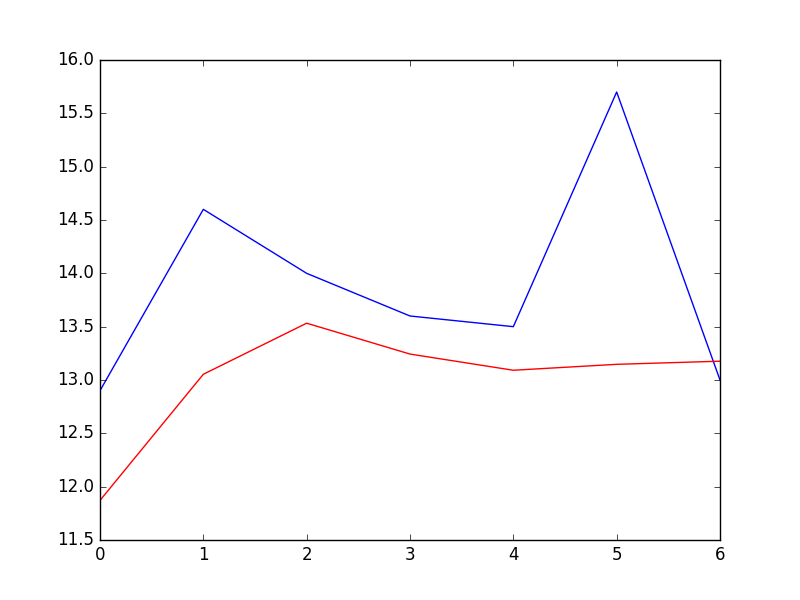固定AR模型的预测

随着新观察结果的出现，statsmodels API无法轻松更新模型。

一种方法是每当新的观察结果可用时重新训练AR模型，并且这可能是有效的方法，如果不是计算上昂贵的话。

另一种方法是使用学习的系数并手动进行预测。这要求保留29个先前观察的历史，并且从模型中检索系数并在回归方程中使用以得出新的预测。

系数以阵列形式提供，截距项后跟每个滞后变量的系数，从t-1到tn开始。我们只需要按照正确的顺序在观察历史中使用它们，如下所示：

yhat = b0 + b1*X1 + b2*X2 ... bn*Xn

以下是完整的示例。

from pandas import Series
from matplotlib import pyplot
from statsmodels.tsa.ar_model import AR
from sklearn.metrics import mean_squared_error
series = Series.from_csv('daily-minimum-temperatures.csv', header=0)
# split dataset
X = series.values
train, test = X[1:len(X)-7], X[len(X)-7:]
# train autoregression
model = AR(train)
model_fit = model.fit()
window = model_fit.k_ar
coef = model_fit.params
# walk forward over time steps in test
history = train[len(train)-window:]
history = [history[i] for i in range(len(history))]
predictions = list()
for t in range(len(test)):
length = len(history)
lag = [history[i] for i in range(length-window,length)]
yhat = coef
for d in range(window):
yhat += coef[d+1] * lag[window-d-1]
obs = test[t]
predictions.append(yhat)
history.append(obs)
print('predicted=%f, expected=%f' % (yhat, obs))
error = mean_squared_error(test, predictions)
print('Test MSE: %.3f' % error)
# plot
pyplot.plot(test)
pyplot.plot(predictions, color='red')
pyplot.show()

再次，运行该示例打印预测和均方误差。

predicted=11.871275, expected=12.900000
predicted=13.659297, expected=14.600000
predicted=14.349246, expected=14.000000
predicted=13.427454, expected=13.600000
predicted=13.374877, expected=13.500000
predicted=13.479991, expected=15.700000
predicted=14.765146, expected=13.000000
Test MSE: 1.451

在比较错误分数时，我们可以看到预测的一个小改进。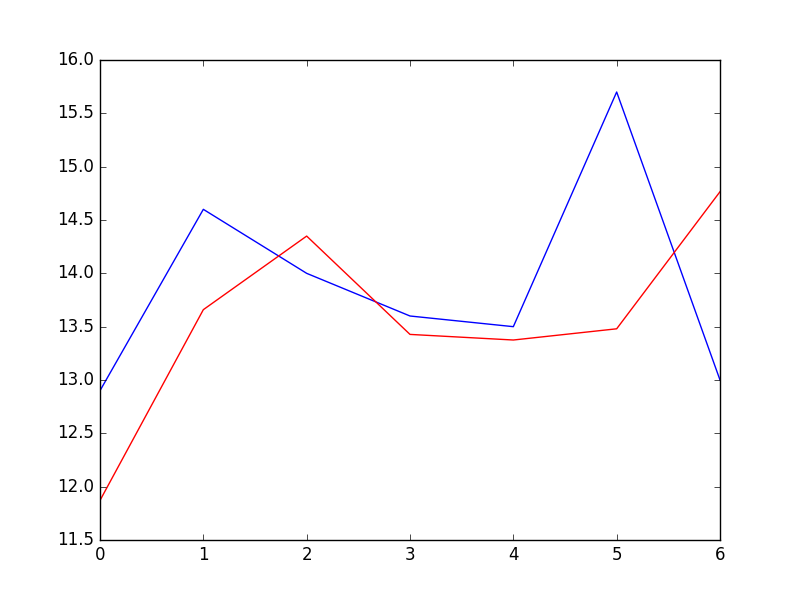滚动AR模型的预测

展开全文• 计划两篇文章介绍如何开发 1D CNN 进行多步时间序列预测。主要内容如下： 如何为单变量数据开发多步时间序列预测的CNN模型； 如何为多变量数据开发多通道多步时间序列预测的CNN模型； 如何为多变量数据开发多头...
• 在本教程中，您将了解如何开发持久性预测，以便Python计算时间序列数据集的性能基准级别。 完成本教程后，您将知道：Python学习交流群：1004391443 计算时间序列预测问题的性能基线的重要性。 如何在Python中...Python
• 2.大多数预测模型都能做时间序列分析（主要是如何将已知问题转化为带有时间戳的序列问题） 参考：如何时间序列转换为Python中的监督学习问题（1） 3.我们常说的预测我总结出来有两层含义： （1）目前我查资料...tensorflow python LSTM
• 时间序列预测可以被视为监督学习问题。 通过重新构建时间序列数据，您可以访问针对您的问题的标准线性和非线性机器学习算法套件。 在这篇文章中，您将了解如何重新构建时间序列问题，作为机器学习的监督学习问题。...
• 如何基于Keras和TensorflowLSTM进行时间序列预测 李倩 发表于 2018-09-06 08:53:16 论智 +关注 编者按：本文将介绍如何基于Keras和Tensorflow，LSTM进行时间序列预测。文章数据来自股票市场数据集，目标是...
• 如何为一步时间序列预测设计一个强大的测试框架。 如何准备数据，开发和评估用于时间序列预测的LSTM递归神经网络。 1. 洗发水销售额数据集； 2. 测试设置； 3. 持续性模型预测； 4. LSTM数据准备； 5. LSTM模型开发...LSTM
• 本篇博客将介绍如何用 keras 深度学习的框架搭建 LSTM 模型对时间序列预测。所用项目和数据集来自：真实业界数据的时间序列预测挑战。 1 项目简单介绍 1.1 背景介绍 本项目的目标是建立内部与外部...
• 基于LSTM的时间序列数据(多步)预测 如何进行多变量LSTM时间序列预测未来一周的数据？ LSTM预测未来一个月航班数 多维多步预测
• Prophet的目的是“使专家和非专家可以更轻松地进行符合需求的高质量预测。 您将学习如何使用Prophet（在Python中）解决一个常见问题：预测下一年公司的每日订单。 数据准备与探索 Prophet...Prophet Python
• 作者：Mohit Sharma翻译：王闯（Chuck)校对：王可汗本文约4100字，建议阅读10+分钟本文介绍了Python进行时间序列分解的不同方法，以及如何在Python中进行时间...大数据 python 机器学习 人工智能 数据分析
• 作者：Mohit Sharma 翻译：王闯（Chuck) 校对：王可汗本文约4100字，建议阅读10+分钟本文介绍了Python进行时间序列分解的不同方法，以及如何在Pytho...大数据 python 机器学习 人工智能 数据分析
• 时间序列预测教程，主要结合Eviews来进行时间序列预测
• 本例展示了如何用LSTM网络预测时间序列数据。为了预测一个序列的未来时间步长值，你可以训练一个sequence-to-sequence LSTM回归网络，其中网络的响应是训练序列值移动了一个时间步长。也就是说，在输入序列的每个...深度学习 LSTM
• 长期以来，我听说时间序列问题只能统计方法(AR，AM，ARMA，ARIMA)。这些技术通常被数学家使用，他们试图不断改进这些技术来约束平稳和非平稳的时间序列。 几个月前，我的一个朋友(数学家、统计学教授...
• 时间序列简单的说就是各时间点上形成的数值序列，时间序列分析就是通过观察历史数据预测未来的值。在这里需要强调一点的是，时间序列分析并不是关于时间的回归，它主要是研究自身的变化规律的（这里不考虑含外生变量...
• RNN进行预测的话，实际上num字段就够了，其他两个字段作为额外的参考信息，读者不妨利用这两条信息构建更复杂的模型，提高预测精度。 接下来我们将多层LSTM 的RNN神经网络去预测这些序列的值，简单来说，我们...python 机器学习 神经网络
• 作者：Jason Brownlee翻译：殷之涵校对：吴振东本文长度为4800字，建议阅读10+分钟本文为大家介绍了如何在Python中使用由Facebook开发的Prophet库进行自动...python 机器学习 人工智能 数据分析 大数据
• 用时间预测分解模型预测商品销量1.1实验目的1.2实验概述1.3 实验目标1.4 实验工具1.5 实验准备实验资源============== 这是一条分割线 ==============必看：想要直接开始操作点击这里 发布本博客皆是为了做笔记，也...
• 作者：ANKIT CHOUDHARY；翻译：王雨桐；校对：丁楠雅；本文约3000字，建议阅读12分钟。本文将通过拆解Prophet的原理及代码实例来讲解如何运用Proph...
• 时间序列预测系列3 主要内容 前2篇已经介绍了基本的LSTM预测模型。本文将引入注意力机制。注意力机制原理本文不进行叙述，本文专注于如何用代码实现。 往往我们预测某一值都是根据前T天的历史数据，将其输入到LSTM......